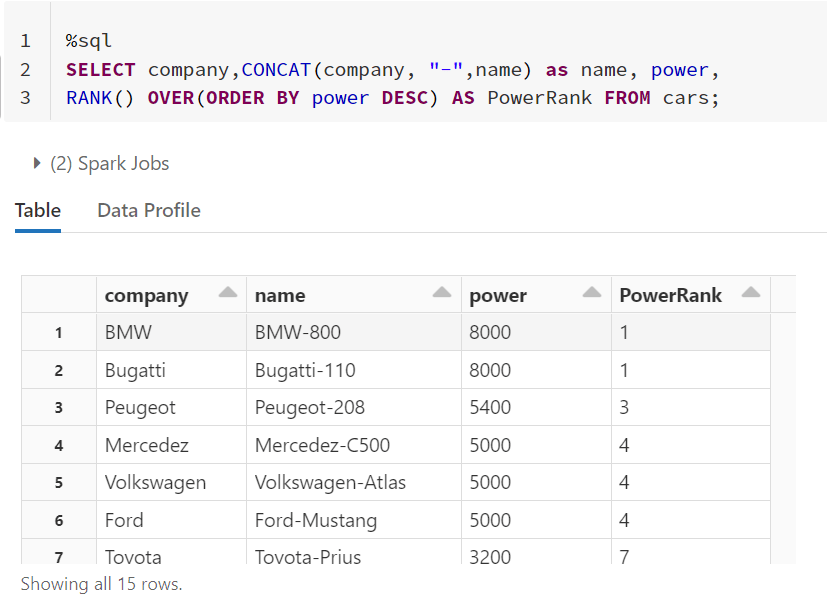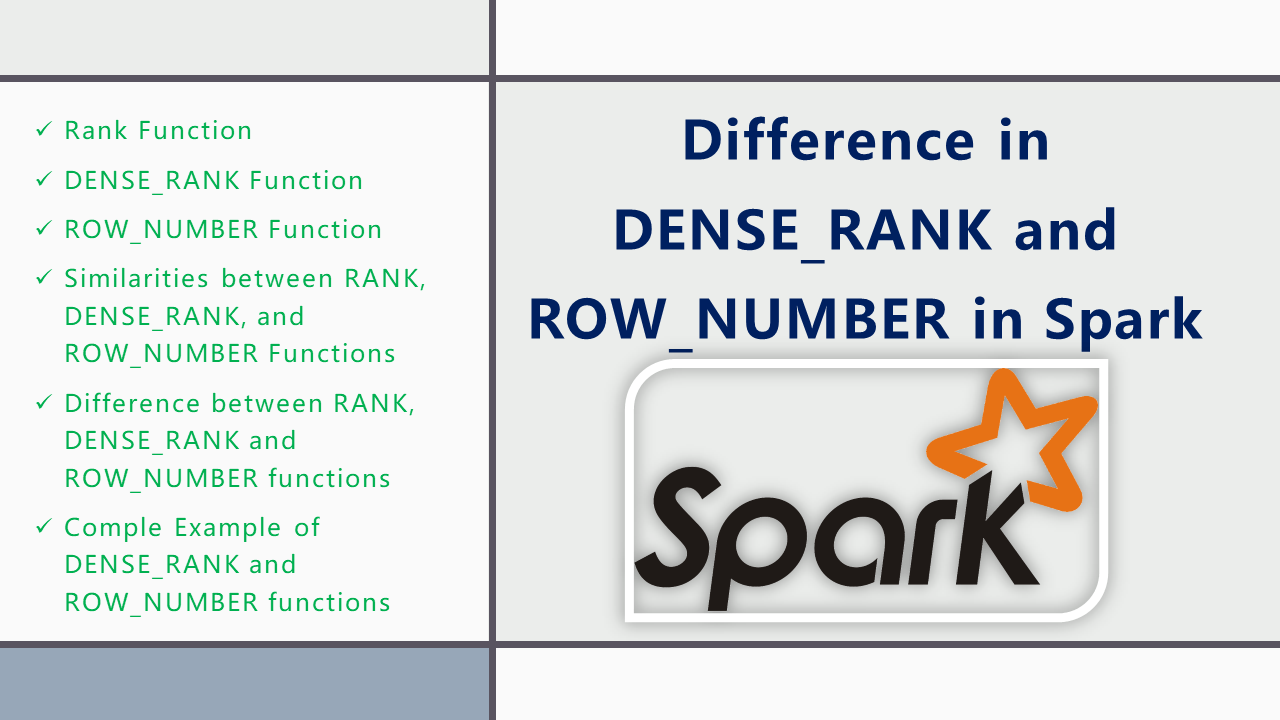# Difference in DENSE_RANK and ROW_NUMBER in Spark

DENSE_RANK and ROW_NUMBER are window functions that are used to retrieve an increasing integer value in Spark however there are some differences between these two. They start with a value based on the condition imposed by the ORDER BY clause. In case of partitioned data, the integer counter is reset to 1 for each partition.

Before jumping into DENSE_RANK and ROW_NUMBER differences, we should be knowing about RANK.

Let’s Create a DataFrame to discuss further. Here, I am using Azure Databricks as my environment hence, I don’t have to create a SparkSession as the Databricks environment provides the `spark` object.

The above sample data has MAX `power` as `8000` for `BWM` and Least `power` `1300` for `Toyota`.

## 1. Rank Function

The RANK is also a window function in Spark that is used to retrieve ranked rows based on the condition of the ORDER BY clause. For example, if you want to find the name of the car with third highest power, you can use `RANK` Function.The script above finds and ranks all the records in the Cars table and orders them in order of descending power. The output looks like this:

The `PowerRank` column in the above table contains the rank of the cars ordered by descending order of their `power`. An interesting thing about the `RANK` function is that if there is a tie between N previous records for the value in the `ORDER BY` column, the `RANK` functions skips the next N-1 positions before incrementing the counter.

For instance, in the above result, there is a tie for the values in the power column between the 1st and 2nd rows, therefore the `RANK` function skips the next (2-1 = 1) one record and jumps directly to the 3rd row.

The `RANK` function can be used in combination with the PARTITION BY clause. In that case, the rank will be reset for each new partition. Take a look at the following script:

In the script above, we partition the results by `company` column. Now for each company, the rank will be reset to 1 as shown above.

## 2. DENSE_RANK Function

The `DENSE_RANK` function is similar to `RANK` function however the `DENSE_RANK` function does not skip any ranks if there is a tie between the ranks of the preceding records. Take a look at the following script.

You can see from the `DensePowerRank` output that despite there being a tie between the ranks of the first two rows, the next rank is not skipped and has been assigned a value of 2 instead of 3.

`PARTITION BY` clause can also be used with the `DENSE_RANK`.

## 3. ROW_NUMBER Function

Unlike the `RANK` and `DENSE_RANK` functions, the `ROW_NUMBER` function simply returns the row number of the sorted records starting with 1. For example, if `RANK` and `DENSE_RANK` functions of the first two records in the `ORDER BY` column are equal, both of them are assigned 1 as their `RANK` and `DENSE_RANK`. However, the `ROW_NUMBER` function will assign values 1 and 2 to those rows without taking the fact that they are equally into account. Execute the following script to see the `ROW_NUMBER` function in action.

From the output, you can see that `ROW_NUMBER` function simply assigns a new row number to each record irrespective of its value.

The `PARTITION BY` clause can also be used with `ROW_NUMBER` function.

### 4. Similarities between RANK, DENSE_RANK, and ROW_NUMBER Functions

The `RANK`, `DENSE_RANK` and `ROW_NUMBER` functions in Spark DataFrame or Spark SQL have the following similarities besides the differences

• All of them require an order by clause.
• All of them return an increasing integer with a base value of 1.
• When combined with a `PARTITION BY` clause, all of these functions reset the returned integer value to 1 as we have seen.
• If there are no duplicated values in the column used by the `ORDER BY` clause, these functions return the same output.

### 5. Difference between RANK, DENSE_RANK and ROW_NUMBER functions

The following are differences between `RANK`, `DENSE_RANK`, and `ROW_NUMBER` functions in Spark

• The `RANK()` function skips the next N-1 ranks if there is a tie between N previous ranks.
• `DENSE_RANK()` function does not skip ranks if there is a tie between ranks
• `ROW_NUMBER()` function has no concern with ranking. It simply returns the row number of the sorted records. Even if there are duplicate records in the column used in the `ORDER BY` clause, the `ROW_NUMBER` function will not return duplicate values. Instead, it will continue to increment irrespective of the duplicate values.

## 6. Comple Example of DENSE_RANK and ROW_NUMBER functions

``````
// Create DataFrame
val data = Seq((1, “Corrolla”, “Toyota”, 1800),
(2, “City”, “Honda”, 1500),
(3, “C200”, “Mercedez”, 2000),
(4, “Vitz”, “Toyota”, 1300),
(5, “Baleno”, “Suzuki”, 1500),
(6, “C500”, “Mercedez”, 5000),
(7, “800”, “BMW”, 8000),
(8, “Mustang”, “Ford”, 5000),
(9, “208”, “Peugeot”, 5400),
(10, “Prius”, “Toyota”, 3200),
(11, “Atlas”, “Volkswagen”, 5000),
(12, “110”, “Bugatti”, 8000),
(13, “Landcruiser”, “Toyota”, 3000),
(14, “Civic”, “Honda”, 1800),
(15, “Accord”, “Honda”, 2000))

val columns = Seq(“id”,”name”, “company”, “power”)
val rdd = spark.sparkContext.parallelize(data)
val df = rdd.toDF(columns:_*)
df.createOrReplaceTempView(“cars”)

// SQL to get dense_rank(), rank() and row_number()
%sql
SELECT company,CONCAT(company, “-“,name) as name, power,
RANK() OVER(ORDER BY power DESC) AS PowerRank,
DENSE_RANK() OVER(ORDER BY power DESC) AS DensePowerRank,
ROW_NUMBER() OVER(ORDER BY power DESC) AS RowRank
FROM cars;
``````

## 7. Conclusion

In this article, I have explained similarities and differences between rank(), dense_rank() and row_number() functions in Spark. rank(), dense_rank() and row_number() functions are used to retrieve an increasing integer value.

### Naveen (NNK)

Naveen (NNK) is a Data Engineer with 20+ years of experience in transforming data into actionable insights. Over the years, He has honed his expertise in designing, implementing, and maintaining data pipelines with frameworks like Apache Spark, PySpark, Pandas, R, Hive and Machine Learning. Naveen journey in the field of data engineering has been a continuous learning, innovation, and a strong commitment to data integrity. In this blog, he shares his experiences with the data as he come across. Follow Naveen @ @ LinkedIn